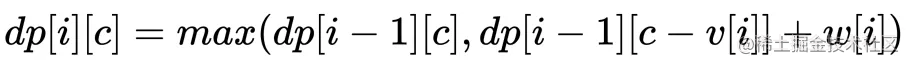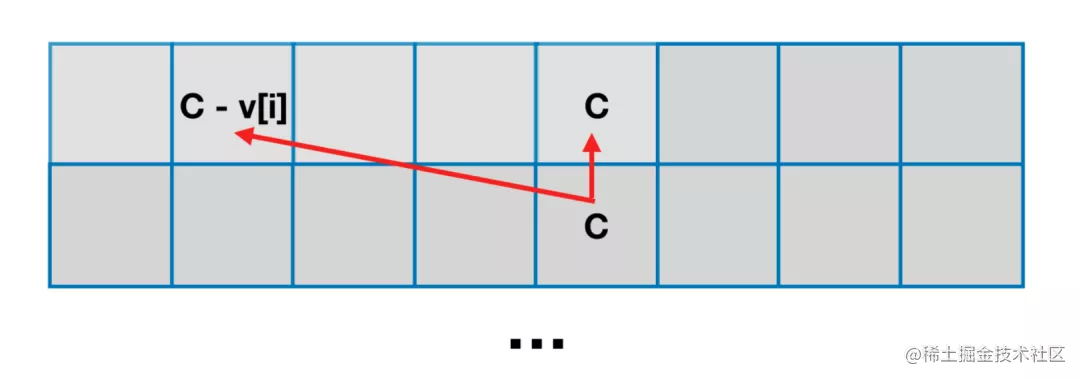# 【背包问题の第一讲】那就从 0-1 背包问题开始讲起吧

## 背包问题本质

「01背包」是指给定物品价值与体积（对应了「给定价值与成本」），在规定容量下（对应了「限定决策规则」）如何使得所选物品的总价值最大。

## 题目描述

$N$ 件物品和一个容量是 $V$ 的背包。每件物品有且只有一件。

$i$ 件物品的体积是 $v[i]$，价值是 $w[i]$

输入: N = 3, C = 4, v = [4,2,3], w = [4,2,3]

输入: N = 3, C = 5, v = [4,2,3], w = [4,2,3]

## dp[N][C+1] 解法

int dfs (int[] v, int[] w, int i, int c);

$i$$c$ 分别代表「当前枚举到哪件物品」和「现在的剩余容量」。

「不选」其实就是 $dp[i - 1][c]$，等效于我们只考虑前 $i - 1$ 件物品，当前容量为 $c$ 的情况下的最大价值。class Solution {
public int maxValue(int N, int C, int[] v, int[] w) {
int[][] dp = new int[N][C+1];
// 先处理「考虑第一件物品」的情况
for (int i = 0; i <= C; i++) {
dp[i] = i >= v ? w : 0;
}
// 再处理「考虑其余物品」的情况
for (int i = 1; i < N; i++) {
for (int j = 0; j < C + 1; j++) {
// 不选该物品
int n = dp[i-1][j];
// 选择该物品，前提「剩余容量」大于等于「物品体积」
int y = j >= v[i] ? dp[i-1][j-v[i]] + w[i] : 0;
dp[i][j] = Math.max(n, y);
}
}
return dp[N-1][C];
}
}

• 时间复杂度：共有 $N * C$ 个状态需要被转移，复杂度为 $O(N * C)$
• 空间复杂度：$O(N * C)$

## dp[C+1] 解法

class Solution {
public int maxValue(int N, int C, int[] v, int[] w) {
int[][] dp = new int[C+1];
// 先处理「考虑第一件物品」的情况
for (int i = 0; i < C + 1; i++) {
dp[i] = i >= v ? w : 0;
}
// 再处理「考虑其余物品」的情况
for (int i = 1; i < N; i++) {
for (int j = 0; j < C + 1; j++) {
// 不选该物品
int n = dp[(i-1)&1][j];
// 选择该物品
int y = j >= v[i] ? dp[(i-1)&1][j-v[i]] + w[i] : 0;
dp[i&1][j] = Math.max(n, y);
}
}
return dp[(N-1)&1][C];
}
}

• 时间复杂度：共有 $N * C$ 个状态需要被转移，复杂度为 $O(N * C)$
• 空间复杂度：$O(C)$

## dp[C+1] 解法class Solution {
public int maxValue(int N, int C, int[] v, int[] w) {
int[] dp = new int[C + 1];
for (int i = 0; i < N; i++) {
for (int j = C; j >= v[i]; j--) {
// 不选该物品
int n = dp[j];
// 选择该物品
int y = dp[j-v[i]] + w[i];
dp[j] = Math.max(n, y);
}
}
return dp[C];
}
}

• 时间复杂度：共有 $N * C$ 个状态需要被转移，复杂度为 $O(N * C)$
• 空间复杂度：$O(C)$

## 背包问题（目录）

1. 01背包 : 本篇

1. 【练习】01背包 : 背包问题 第二讲

2. 【学习&练习】01背包 : 背包问题 第三讲

2. 完全背包 : 背包问题 第四讲

1. 【练习】完全背包 : 背包问题 第五讲

2. 【练习】完全背包 : 背包问题 第六讲

3. 【练习】完全背包 : 背包问题 第七讲

3. 多重背包 : 背包问题 第八讲

4. 多重背包（优化篇）

1. 【上】多重背包（优化篇）: 背包问题 第九讲

2. 【下】多重背包（优化篇）: 背包问题 第十讲

5. 混合背包 : 背包问题 第十一讲

6. 分组背包 : 背包问题 第十二讲

1. 【练习】分组背包 : 背包问题 第十三讲
7. 多维背包

1. 【练习】多维背包 : 背包问题 第十四讲

2. 【练习】多维背包 : 背包问题 第十五讲

8. 树形背包 : 背包问题 第十六讲

1. 【练习篇】树形背包

2. 【练习篇】树形背包

9. 背包求方案数

1. 【练习】背包求方案数
10. 背包求具体方案

1. 【练习】背包求具体方案
11. 泛化背包

1. 【练习】泛化背包